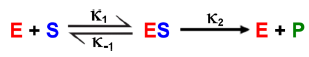Clutch Prep is now a part of Pearson
Ch. 6 - Enzymes and Enzyme KineticsWorksheetSee all chapters

# Rate Constants and Rate Law

See all sections
Sections
Enzymes
Enzyme-Substrate Complex
Lock and Key Vs. Induced Fit Models
Optimal Enzyme Conditions
Activation Energy
Types of Enzymes
Cofactor
Catalysis
Electrostatic and Metal Ion Catalysis
Covalent Catalysis
Reaction Rate
Enzyme Kinetics
Rate Constants and Rate Law
Reaction Orders
Rate Constant Units
Initial Velocity
Vmax Enzyme
Km Enzyme
Michaelis-Menten Assumptions
Michaelis-Menten Equation
Lineweaver-Burk Plot
Michaelis-Menten vs. Lineweaver-Burk Plots
Shifting Lineweaver-Burk Plots
Calculating Vmax
Calculating Km
Kcat
Specificity Constant

Concept #1: Introduction to Rate Constants

Concept #2: Types of Rate Constants

Practice: Which of the following rate constants is negligible for the initial velocity (V 0) of an enzyme-catalyzed reaction?

Concept #3: Rate Law: Calculating Reaction Rate (v) with Rate Constant (k)

Practice: Calculate the reaction rate for the following simple reaction if k = 1.3 x 10 -1 M-1s-1, initial [A] = 4.0 x 10-3 M, and the initial [B] = 6.0 x 10 -3 M (for simple reactions, assume coefficients are reaction orders):

A + B → 2C

Concept #4: Rate Laws for Enzyme-Catalyzed Reactions

Practice: Write out the rate law equations for each association/dissociation indicated below.a) Rate law for ES dissociation into E + P: V = ______________

b) Rate law for E + S association: V = ______________

c) Rate law for ES dissociation back into E + S: V = ______________

Practice: In a typical enzyme-catalyzed reaction, when & why is the rate constant k  -2 negligible?